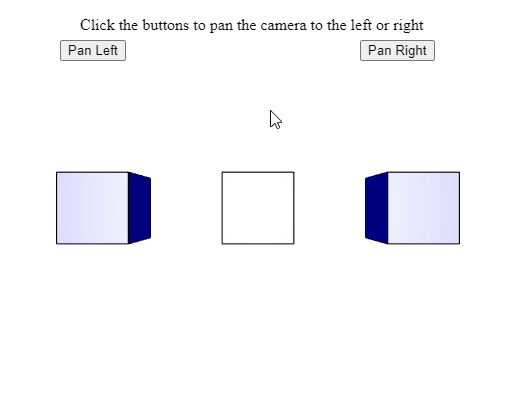Related Articles

# p5.Camera pan() Method

• Last Updated : 28 Jul, 2020

The pan() method of p5.Camera in p5.js is used to rotate the view, that is, pan the camera according to the given amount of rotation. The camera can be panned both to the left or right by rotating the camera anticlockwise or clockwise.

Syntax:

```pan( angle )
```

Parameters: This method accepts a single parameter as mentioned above and described below:

• angle: It is a number that denotes the amount that the camera has to rotate. The units of rotation to be used can be specified using the angleMode() method. A value greater than 0 would rotate the camera counterclockwise, that is, pan the camera to the left. Similarly, for negative values, the camera would rotate in the clockwise direction.

The example below illustrates the pan() method in p5.js:

Example:

## Javascript

 `let currCamera;`` ` `function` `setup() {``  ``createCanvas(500, 400, WEBGL);``  ``helpText = createP(``    ``"Click the buttons to pan the camera "` `+``    ``"to the left or right"``);``  ``helpText.position(80, 0);`` ` `  ``currCamera = createCamera();`` ` `  ``// Set the angle mode in degrees``  ``angleMode(DEGREES);`` ` `  ``// Create the buttons for panning the camera``  ``newCameraBtn = createButton(``"Pan Left"``);``  ``newCameraBtn.position(60, 40);``  ``newCameraBtn.mouseClicked(panCameraLeft);`` ` `  ``newCameraBtn = createButton(``"Pan Right"``);``  ``newCameraBtn.position(360, 40);``  ``newCameraBtn.mouseClicked(panCameraRight);``}`` ` `function` `panCameraLeft() {`` ` `  ``// Pan the camera to the left``  ``// that is, rotate counterclockwise``  ``// using a value greater than 0``  ``currCamera.pan(10);``}`` ` `function` `panCameraRight() {`` ` `  ``// Pan the camera to the right``  ``// that is, rotate clockwise``  ``// using a value less than 0``  ``currCamera.pan(-10);``}`` ` `function` `draw() {``  ``clear();``  ``lights();``  ``specularMaterial(``'blue'``);`` ` `  ``// Create three boxes at three positions``  ``translate(-150, 0);``  ``box(65);``  ``translate(150, 0);``  ``box(65);``  ``translate(150, 0);``  ``box(65);`` ` `  ``// Draw 2 spheres only visible after``  ``// panning left and right``  ``translate(0, 0, 250);``  ``sphere(30);``  ``translate(-300, 0, 0);``  ``sphere(30);``}`

Output:Online editor: https://editor.p5js.org/

My Personal Notes arrow_drop_up